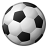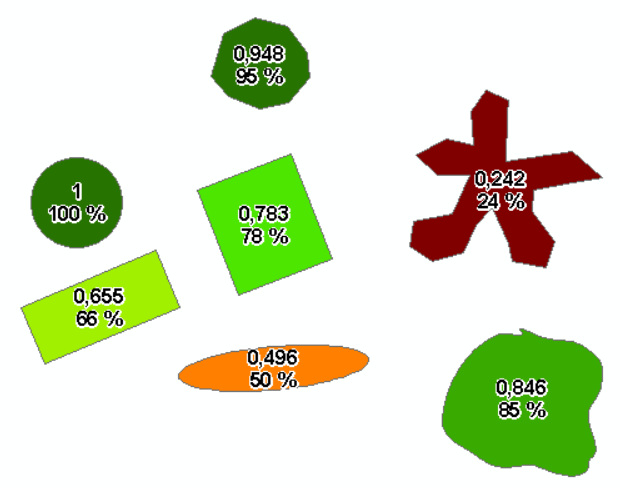# Is there a way to calculate the roundness of a polygon?

3052
3
05-03-2018 06:03 AMNew Contributor III

Is there a way to calculate the roundness of a polygon?

3 RepliesbyEsri Esteemed Contributor

There is an interesting discussion here: vector - Calculating roundness/compactness of polygon? - Geographic Information Systems Stack Exchan...  and some more background here: Roundness (object) - Wikipedia .

Is that what you are looking for?byMVP Legendary Contributor

'circularity'  various definitions: aka roundness

You can calculate some of the volume properties using

Bounding Containers ArcMap has an equivalent toolset  (ie convex hull, MABE, aligned rectangle, minimum area circle)

Minimum Area Bounding Ellipse

Concave Hull  The ratio of the convex hull to the concave hull is often a measure of container tortuosity.byEsri Esteemed Contributor

An example of what you get when  you execute this field calculation using the Python parser:

``4 * math.pi * !SHAPE_Area!  / !SHAPE_Length!**2‍``